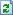Sunday, 2019-03-24, 3:51 PM
Welcome Guest | RSS
Tag Board
 --15 sec30 sec1 min2 min500Our poll Which .Net class is more interset for you ? I like most C#.Net I like most ASP.Net I like both C# & ASP in equal Total of answers: 219
 StatisticsTotal online: 1 Guests: 1 Users: 0

03-01-2011

Polymorphism: Polymorphism is one name many forms.

Def: "Implementing multiple functionality with the same name can be called as polymorphism that means implementing multiple functions with same name can be called as polymorphism"

We can achieve polymorphism in 2 ways.
2. Function overriding

1. Function overloading: Function overloading means having multiple methods with the same name but a different number of arguments or different type of arguments in a single class or a combination of base & derived class.

{
Public class calculate
{
Public int add(int a, int b)
{
return a + b;
}
Public int add(int a , int b, int c)
{
return a+b+c;
}
Public double add(double a, double b)
{
return a + b;
}
Public double add(double a , double b, double c)
{
return a+b+c;
}

Class mainclass
{
Void main()
{
Calculate cal = new calculate();
int res1 = cal.add(10, 20);
Console.WriteLine("addition of two integers is " +res1);
int res2 = cal.add(10, 20, 30);
Console.WriteLine("Addition of 3 integers is " +res2)
double res3 = cal.add(10.4, 20.5);
Console.WriteLine("addition of two double is " +res3);
int res4 = cal.add(10.4, 20.5, 30.5);
Console.WriteLine("addition of 3 double is " +res4);
}
}
}
Output: addition of two integer is 30
addition of 3 integer is 60
addition of 2 double is 30.9
addition of 3 double is 61.4

Function overloading will depend on below 3 points
1. Type of parameter to a function
2. Number of parameter to a function
3. Order of parameter to a function

Function overloading will not depend on below two points.
1. return type of a function.
2. Name of parameter to a function

1. Type of parameter to a function:
namespace typeofparameterexample
{
Public class myclass
{
Public void print(int a)
{
Console.WriteLine("a value is " +a);
}
Public void print(String s)
{
Console.WriteLine("S avalue is " +s);
}

Class mainclass
{
Void main()
{
myclass mc = new myclass();
mc.print(10);
mc.print(Sathya);
}
}
}
Output: a value is 10
s value is Sathya.

 Search

 Time

Calendar

 «  March 2019  » Su Mo Tu We Th Fr Sa 1 2 3 4 5 6 7 8 9 10 11 12 13 14 15 16 17 18 19 20 21 22 23 24 25 26 27 28 29 30 31